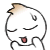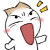#www.vustudents.ning.com

We non-commercial site working hard since 2009 to facilitate learning Read More. We can't keep up without your support. Donate.

 Assignment No. 02 Semester: Fall 2014 Compiler Construction CS606 Total Marks: 20   Due Date: 08/12/2014 Objective:   To learn and understand basic concepts of Parse Trees, Top Down Parsers, Predictive Parsing, First and Follow Sets in building a Lexical analyzer. Instructions: It should be clear that your assignment will not get any credit (zero marks will be awarded) if:   The assignment is submitted after due date. The submitted assignment does not open or file corrupt. The assignment is copied (from other student or copy from handouts or internet). Student name and ID are not mentioned in the assignment file. It is in some format other than .doc or .docx(MS Word Document). For any query about the assignment, contact at cs606@vu.edu.pk Question No 1:                                                                                                                       Marks 10 Consider the following grammar; you are required to write left most derivation of the string “aebb”.             Question No 2:                                                                                                                        Marks 10              Consider the following grammar for arithmetic expressions:   A à id X X àA Y |ε Y à- X | + X | / X | * X   Show the first set for the non-terminals X and Y in the grammar. BEST OF LUCK

Views: 1801

### Replies to This Discussion

Ik qareeb tareen example:

Question:

S → ABC | BC
A → aA | a
B → b | ε
C → c | d | ε

first(A) = first(aA) ∪ first(a)
= {a} ∪ {a}
= {a}
first(B) = first(b) ∪ first(ǫ)
= {b} ∪ ∅
= {b}
first(C) = first(c) ∪ first(d) ∪ first(ǫ)
= {c} ∪ {d} ∪ ∅
= {c, d}
first(S) = first(ABC) ∪ first(BC)
= (first(A) ∪ ∅) ∪ (first(B) ∪ first(C) ∪ ∅)
= {a} ∪ {b} ∪ {c, d}
= {a, b, c, d}

T = { number, id, +, -}

yh chyzain terminal main ay gi

bs is kyHisab Sy bana daain

InshaAllah marks mily gain:P

Reply by مَلیکا ایمان 4 minutes ago

then iska First just {a,b} hona chahiaye na k  {a,b,c,d,e}..Malika Api

a,b tu hn pr Agy 2nd main dekho A hy nonterminal us ky andr phr XYZ hn

Nonterminal tu isly wOo c,d,e aarahy hain samj aa gi hu gi abCapital Letters are Non Terminal, However, small and symbols are Terminal

So here, A, Y,X are Nonterminal and +,-,*,/ are the Terminal..

it means,

First(X)={,ε}

First(Y)={-,+,/,*}

right??

ys nic approach,

bt id iz also terminal. :)

Second qustion,

acording to Lec 18,

first of al v hav to write terminals,

which r +, -, / , id

Then use nonterminals by using algorithm

For using algo, we hav to start with FIRST(A) whch is equal to idX

then choose right most nonterminal wch is X then use this n find the nonterminals of X wch is AY now take frst alphabet n see alphabtes of A if there is no any Epsilon then stop here.

Go nxt n use this algo again till u find Epsilon.

forexampl;

FIRST(A) = FIRST(A) - epsilon.

i got 18 marks

*******************

mistake was id is missing from first X.same mistake ..

hmm good appiapi id bi ana tha wo bi terminal main hi ana thamri tu yh book clr A hy .

welcomeCongrats